# 牛顿冷却系数选择并评价广告渠道策略及用户访问次数预估（上）

wuchangjian2021-11-04 10:03:40编程学习

``import pandas as pd``import numpy as np``df = pd.read_excel(r"C:\Users\dai\Desktop\序列行为.xlsx")``df.head()``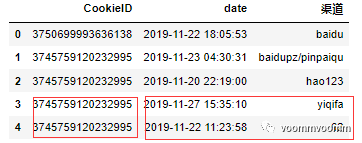``len(df)  #271``df.info()``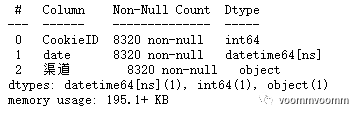``def get_interview(time2,time1):``    date2 = time2``    date1 = time1``    seconds = (date2 - date1).total_seconds() #秒``    minutes = (date2 - date1).total_seconds()/60 #分``    hours = (date2 - date1).total_seconds()/3600 #小时``    days = (date2 - date1).total_seconds()/3600/24 #天``    return seconds,minutes,hours,days``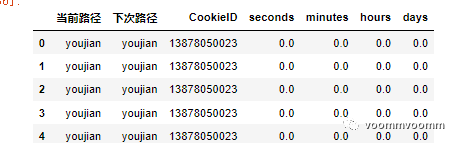``def cut_column(df):``    df2 = pd.DataFrame()``    id_list = pd.Series(np.unique(df["CookieID"])).to_list()``    for i in id_list:``        data_cut = pd.DataFrame()``        data = df[df["CookieID"]==i]``        data = data.sort_values("date")``        data = data.reset_index(drop=True)``        length = len(data)``        way_now = []``        way_next = []``        seconds = []``        minutes = []``        days = []``        hours = []``        if length==1:``            way_now.append(data.loc[0,"渠道"])``            way_next.append("just_one")``            seconds.append(0)``            minutes.append(0)``            hours.append(0)``            days.append(0)``        elif length==2:``            way_now.append(data.loc[0,"渠道"])``            way_next.append(data.loc[1,"渠道"])``            seconds.append(get_interview(data.loc[1,"date"],data.loc[0,"date"]))``            minutes.append(get_interview(data.loc[1,"date"],data.loc[0,"date"]))``            hours.append(get_interview(data.loc[1,"date"],data.loc[0,"date"]))``            days.append(get_interview(data.loc[1,"date"],data.loc[0,"date"]))           ``        else:``            row_index= 0``            while row_index<length-1:``                way_now.append(data.loc[row_index,"渠道"])``                way_next.append(data.loc[row_index+1,"渠道"])``                seconds.append(get_interview(data.loc[row_index+1,"date"],data.loc[row_index,"date"]))``                minutes.append(get_interview(data.loc[row_index+1,"date"],data.loc[row_index,"date"]))``                hours.append(get_interview(data.loc[row_index+1,"date"],data.loc[row_index,"date"]))``                days.append(get_interview(data.loc[row_index+1,"date"],data.loc[row_index,"date"]))``                row_index+=1``        data_cut["当前路径"] = way_now ``        data_cut["下次路径"] = way_next``        data_cut["CookieID"] = pd.Series(np.unique(df["CookieID"])).to_list()``        data_cut["seconds"] = seconds          ``        data_cut["minutes"] = minutes                ``        data_cut["hours"] = hours``        data_cut["days"] = days``        df2= df2.append(data_cut) ``        df2 = df2.reset_index(drop=True)``        return df2``df2 = cut_column(df)``len(df2) #255``df2.head()``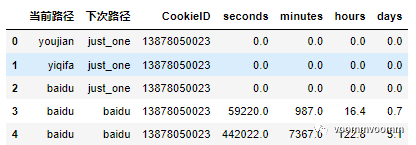``df2.describe([0.01,0.05,0.1,0.25,0.5,0.75,0.9,0.95,0.97,0.98])``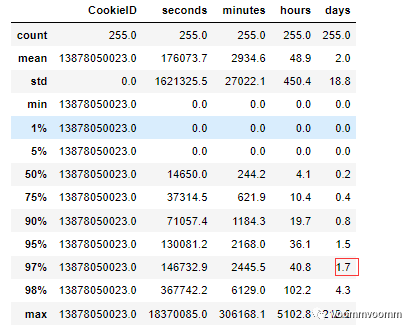````df2 = df2[df2["days"]<1.71]``len(df2) #247，只减少了8个值``import seaborn as sns``sns.distplot(pd.DataFrame(pd.Series(np.round(df2["hours"]))).reset_index(drop=True)["hours"])`
```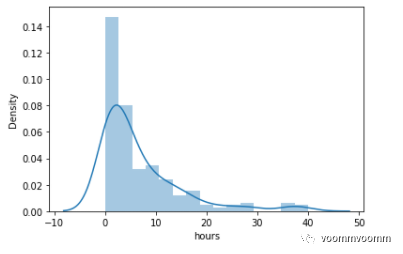``date_max = np.max(df["date"])``df["minus"] = date_max - df["date"]``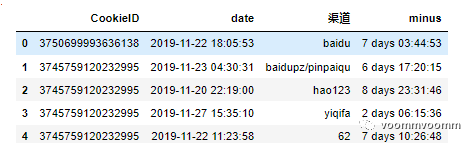``hour = df["minus"].apply(lambda x:str(x).split(" ")).apply(lambda x:str(x).split(":"))``day = df["minus"].dt.days``hour = hour.apply(lambda x:int(x))``hour_to_day = hour/24``day_fina= day+hour_to_day``df["day_fina"] = day_fina``

百度的初始得分 = 百度的各次访问间隔时长*冷却系数

360的初始得分 = 360的各次访问间隔时长*冷却系数

hao123的初始得分 = hao123的各次访问间隔时长*冷却系数

所有渠道初始得分 = 百度的初始得分+360的初始得分+hao123的初始得分

百度的最终得分 =百度的初始得分/所有渠道初始得分，360与hao123类似。

因为百度访问了两次，所以百度的得分会更高，为360/hao123 的两倍。

百度的最终得分 = 1

``df_orginal = df.sort_values("date",ascending=False)``df_orginal = df_orginal.drop_duplicates(subset=["CookieID","渠道"],keep="first").reset_index(drop=True)``df_orginal.head(10)``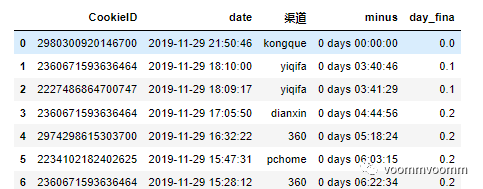``import math ``from matplotlib import pyplot as plt``plt.style.use('fivethirtyeight')``number = np.max(df_unique["minus"].value_counts().index)``for i in np.linspace(3.2, 3.3, num=10):``    y_list = []``    x_list = [] ``    for x in np.linspace(0, 1.7, num=20):``        y = 1/math.exp(i * x)``        y_list.append(y)``        x_list.append(x)``    print(y)``    print(i)``    plt.figure(figsize=[20,5])``    plt.plot(x_list,y_list)``    plt.show()``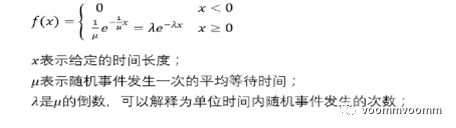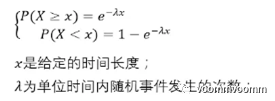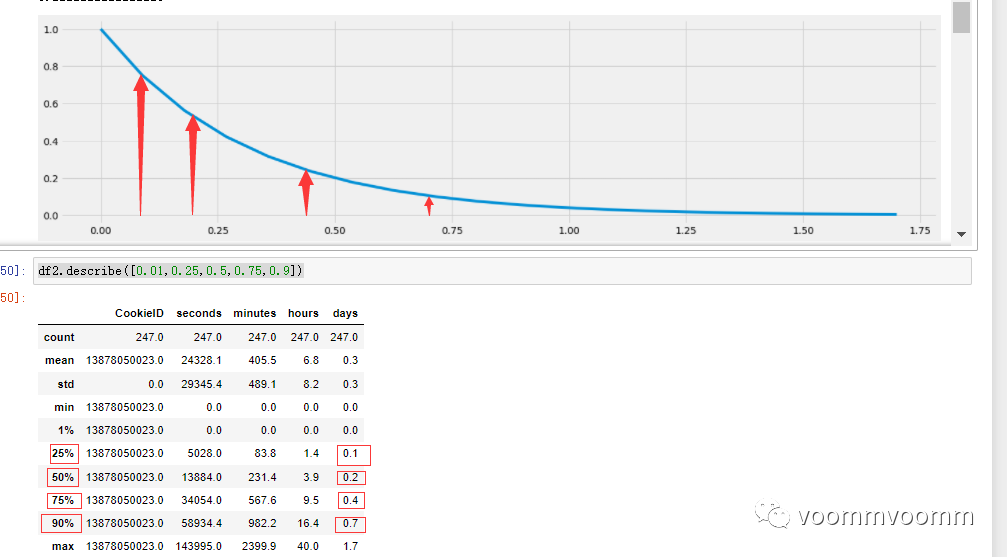``df_orginal["score"] = df_orginal["day_fina"].map(lambda x:1/np.exp(3.222 * x)) ``df_orginal``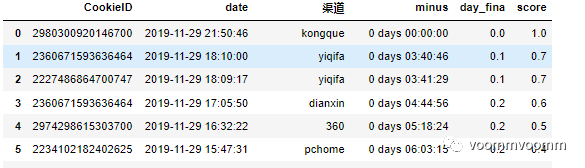``pd.DataFrame(df_orginal.groupby("渠道")["score"].sum()).reset_index().sort_values("score",ascending=False)``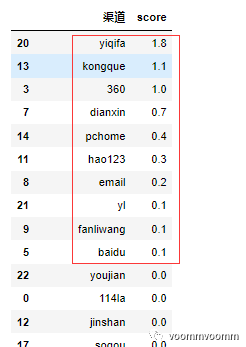``pd.DataFrame(df_orginal.groupby("渠道")["CookieID"].count()).reset_index().sort_values("CookieID",ascending=False)``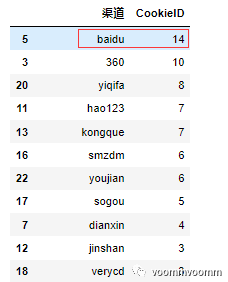``cookieid_all=len(df_orginal["CookieID"])``day_width = []``cookid_number = []``print(cookieid_all)``for x in np.linspace(0, 3, num=20):``    y = cookieid_all/math.exp(3.222 * x)``    day_width.append(x)``    cookid_number.append(y)``plt.figure(figsize=[20,5])``plt.plot(day_width,cookid_number)``plt.show()``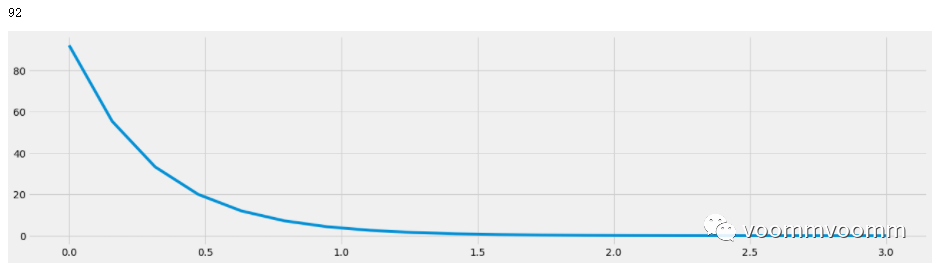``time =np.linspace(0,7,70) #访问时间``view_number =np.random.randint(1500,2000,size=(70,))  #访问用户数``data = pd.DataFrame({"time":time,"view_number":view_number})``data.head()``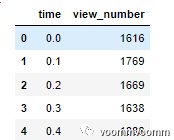``data["score"] = data["time"].map(lambda x:1/np.exp(3.222 * x)) ``for i in np.arange(len(data)):``    while i<len(data)-1:``        data.loc[i+1,"score2"] = data.loc[i,"score"] - data.loc[i+1,"score"]``        i=i+1`` data = data.fillna(0)`` data.head()``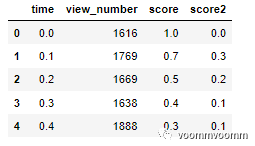``result= []  ``for loop_ in np.arange(len(data)):``    view_remain = 0``    i = 0``    for number_ in data["view_number"][0:loop_+1]:``        calculate = data.loc[loop_-i,"score2"] * number_``        i=i+1``        view_remain = view_remain+calculate``    result.append(view_remain)``result``

result代表的就是当前时间段有多少次访问是受到上一时间段的影响才在本次进行了访问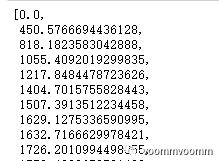``data["real_new"] = data["view_number"] -data["result"]``plt.plot(data.time,data.real_new)``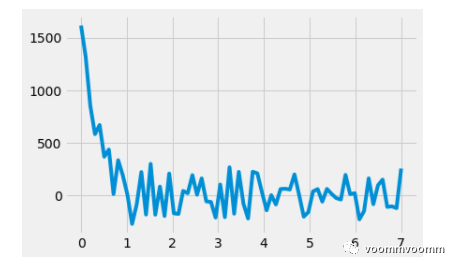1、单渠道选择

2、用户访问次数预估

3、真实渠道效果在各时间段的评价

...

### 威海市原副市长周永迪被“双开”，长期任职城建领域

2022-08-24 13:05:10 据山东省纪委监委8月24日通报...

...

...

### 欢迎大学生！湖南一高校开启新生报到模式

2022-08-28 17:25:10 又是一年迎新季。莘莘学子从高中...

### 大数据解析：程序员到底能干到多少岁？

...

#### 发表评论◎欢迎参与讨论，请在这里发表您的看法、交流您的观点。